﻿ Dirac's electron spin g-factor

# Electron spin g factor of Dirac equation.

## Getting electron g-factor from Dirac equation.

### [ "Bohr magneton" originates in Bohr model. ]

It is said that if we use Dirac equation, we can get the electron spin g factor 2 "naturally".
(And using the renormalization theory of the quantum electrodynamics (QED), we can correct its value a little. But this QED correction is also based on Dirac's value 2. )

(Fig.1) Electron spin (= Bohr magneton ) = circular movement (= Bohr magneton ) ?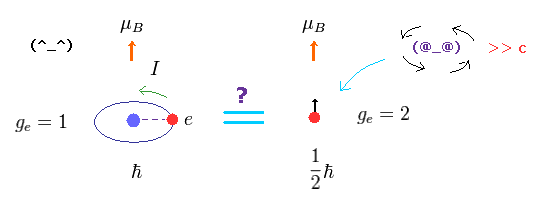Surprisingly, the magnetic moment of the electron spin is just equal to that of Bohr's circular orbit (= Bohr magneton ).
As shown on this page, if we use Bohr model, its g-factor becomes 1 and its minimum angular momentum becomes ħ (not 1/2 ħ).

So its magnetic moment which can be measured in the experiments doesn't change. (= g-factor × angular momentum = 1 × 1 = 2 × 1/2 = magnetic moment. )
In Bohr's orbit, the magnetic moment means the current I × the area of circular orbit.

## G-factor "2" is NOT a natural result.

(Fig.2) G-factor is a natural result ?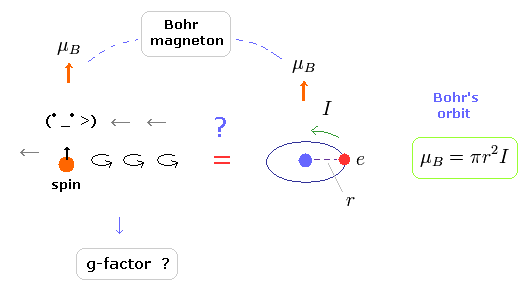The penning trap can measure directly this magnetic moment. So only the interpretaion of g-factor changes.
Furthermore, we don't have to be annoyed by the strange phenomena such as faster-than-light spinning speed and two-valued nature of electron spin in Bohr model.

By the way, the electron spin g factor 2 is really the "natural" result of Dirac equation ?
And is it limitted only to 2 ? ( Why the electron g-factor can not be 1.7, 2.3 or 3.4 ?? )

Here we try to get the electron spin g-factor 2 by Dirac equation concretely.
The important point is that the electron magnetic moment (= Bohr magneton ) originates in Bohr's orbit.

## Derivation of Dirac spin g factor "2".

(Eq.1)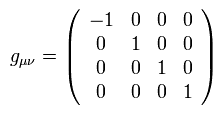And γ matrices are
(Eq.2)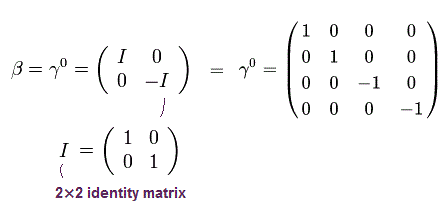" I " means 2 × 2 identity matrix.
And
(Eq.3)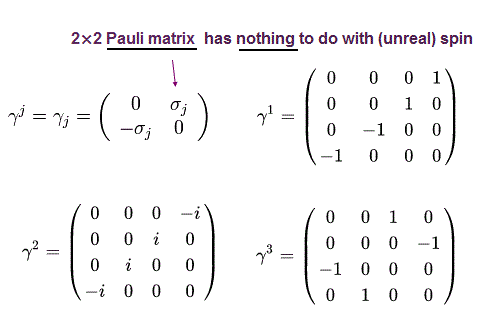where σj mean 2 × 2 Pauli matrices of
(Eq.4)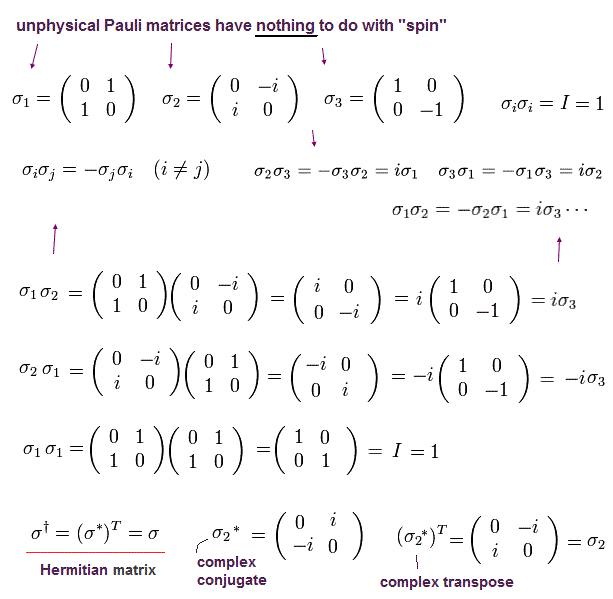(Eq.5)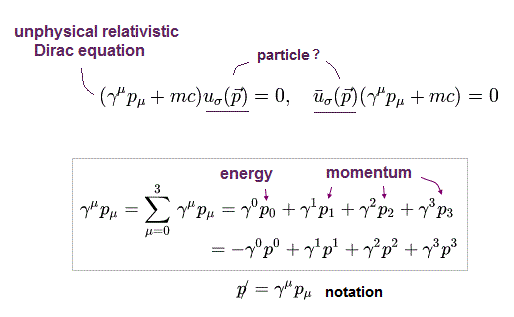Using the upper γ matrices, we have
(Eq.6)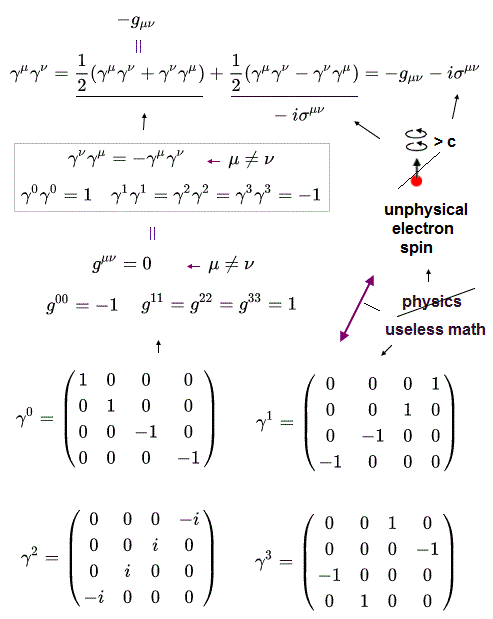σ of Eq.6 is defined by, (This σ is 4 × 4 matrix. )
(Eq.7)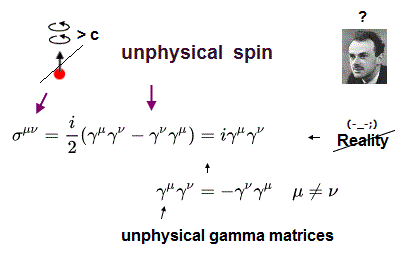This is 4 × 4 spin form.
As you notice, this spin matrices include "zero" components, from which we can NOT get the usual magnatic moment.
( Because "electric" potential and "magnetic" potential are the same degree. )
But they try to use this form as "spin".

Here we use
(Eq.8)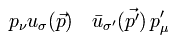Multiplying Eq.6 by the first operator of Eq.8 from the right.
(Eq.9)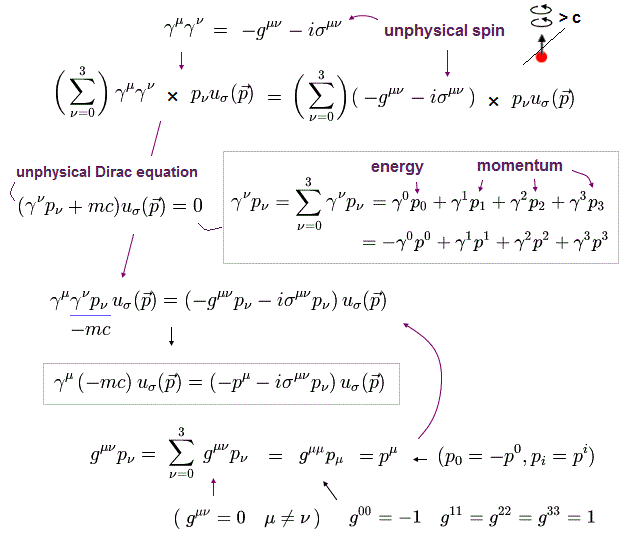where Eq.5 is used.

Multiplying Eq.6 by the second operator of Eq.8 from the left.
(Eq.10)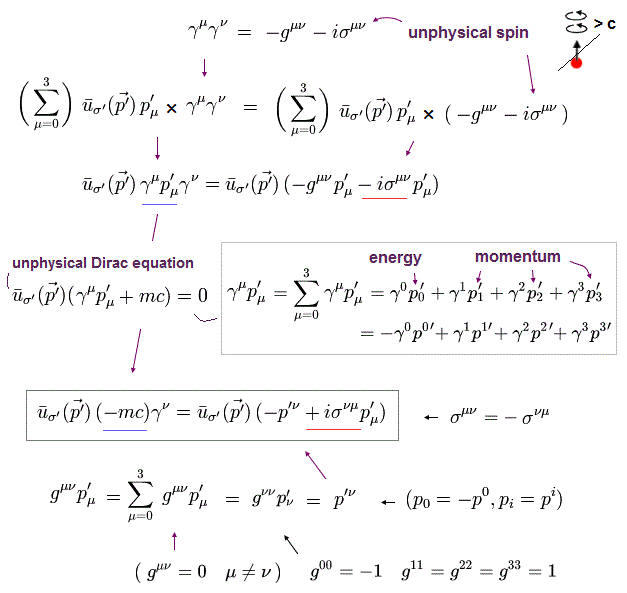The last term uses antisymmetric property of σ ( see Eq.7 ).

And dividing the both sides of Eq.9 by -mc
(Eq.11)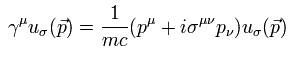Exchanging the variables μ and ν of Eq.10, and dividing it by -mc,
(Eq.12)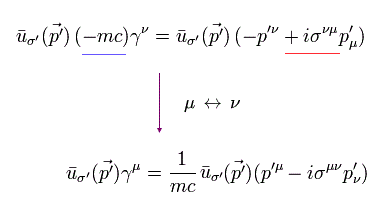Multiplying Eq.11 by the first operator of Eq.13 from the left, and multiplying Eq.12 by the second operator of Eq.13 from the right.
(Eq. 13)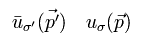And then summing these two results, and dividing the sum of them by 2, we can get
(Eq.14)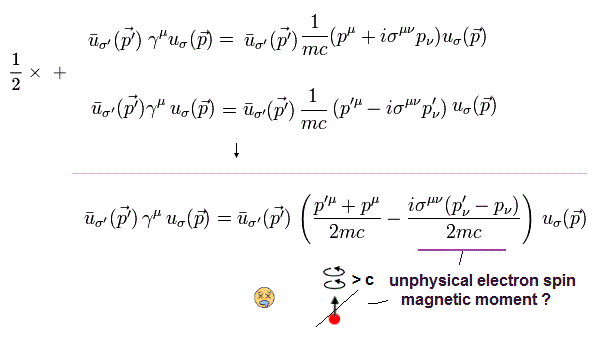where
(Eq.15)This "q" is ( virtual ) photon = external magnetic field.
According to this definition, when the electron's momentum (= velocity ) is constant ( p'-p = 0 ), external magnetic field is zero, even if it exists.
This is strange.

## "Artificial" g factor.

Using the relation p' - p = q and defining c = ħ = 1, Eq.14 becomes
(Eq.16)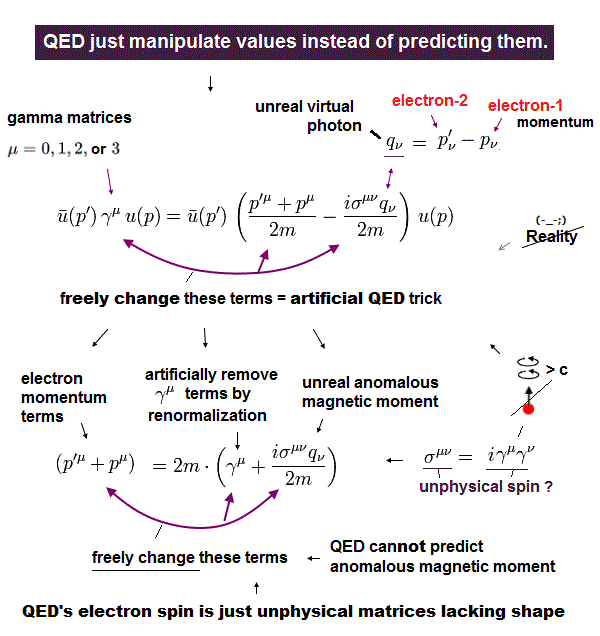Eq.16 is used in calculating g-factor.
As shown on this page, vector potential A includes exponential function, so we obtain the spin-magnetic field interaction from Eq.7 and Eq.16, as follows,
(Eq.17)As you notice, we define spin angular momentum is "1/2", so electron g-factor (= ge ) becomes "2".
So g-factor "2" is not a natural thing.
To agree with "Bohr magneton", it becomes 2.

Bohr magneton is
(Eq.18)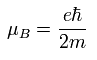"z" component of spin is
(Eq.19)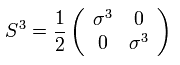Even in Schrodinger's form, the quantum mechanics uses the mathematical tricks like ( See this page. )
(Eq.20)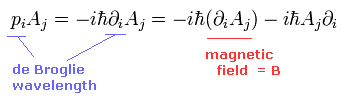where p (= electron's momentum ) includes derivative, which acts on before and after Aj.
So de Broglie wavelength of electron is changed into derivative of the vector potential A to get the magnetic field.
This is strange.

## Time evolution and interaction in QED.

### [ Heisenberg picture ]

In this section, we explain about the time evolution and interaction used in quantum electrodynamics.
As you know, the time dependent Schrodinger equation is expressed as
(Eq.21)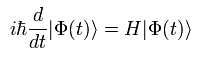So the eigenstates ( Φ(t) ) of Schrodinger pictures contain the time variable (= t).

The initial state ( t=0 ) is defined as
(Eq.22)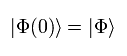So when the Hamiltonian (H) is time independent, the solution of Eq.21 is
(Eq.23)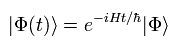The quantum field theory (QFT) often uses Heisenberg pictures instead of Schrodinger pictures.
Because the main places of renormalization are operators rather than eigenstates.
(The eigenstates themselves are not very important in QFT and QED.)

So the operators ( A(t) ) are time dependent in Heisenberg pictures, as follows,
(Eq.24)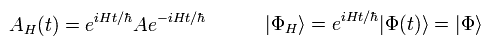( Instead, the eigenstates (Φ) are time independent in Heisenberg pictures. )

From Eq.24, we can know the A(t) of Eq.24 satisfies the following Heisenberg equation of motion.
(Eq.25)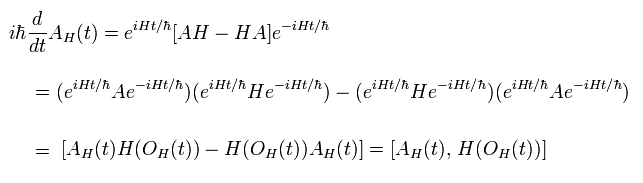where each operator ( OH(t) ) in Hamiltonian is expressed like Eq.24.

### [ Interaction picture ]

Hamiltonian can be divided as
(Eq.26)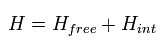which mean free energy term and interaction term, respectively.

Here we define the operators of interaction pictures as follows,
(Eq.27)The important part in QED is the interaction term, so we suppose the eigenstates evolve by the interaction Hamiltonian, as follows,
(Eq.28)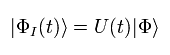where U(t) is
(Eq.29)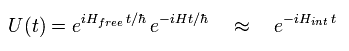U(t) is called the interaction picture propagator or time-evolution operator.
The conjugate operator of Eq.29 is
(Eq.30)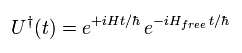So U U = 1. This means that U(t) is the unitary operator.

The interaction picture operator AI(t) of Eq.27 is expressed using Heisenberg picture operator AH(t) of Eq.24 and U(t) of Eq.29 and 30, as follows,
(Eq.31)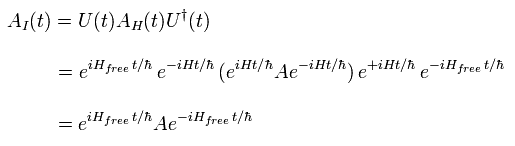### [ Solution of U(t) ]

We have to know more concrete expressions of U(t) than Eq.29 for perturbation calculation of electron g-factor.
From Eq.29, the simple differential equation of U(t) is
(Eq.32)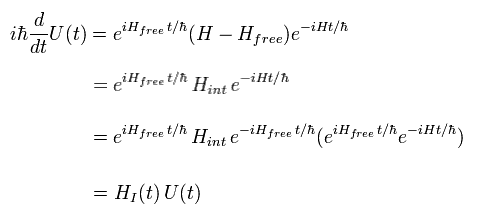where the initial state is U(0) = 1.

The meaning of Eq.32 is equal to
(Eq.33)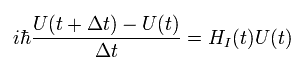So,
(Eq.34)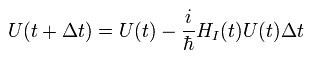Multiplying Eq.34 by U of Eq.30 from right side,
(Eq.35)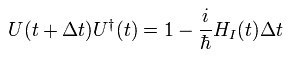Here we can define as follows,
(Eq.36)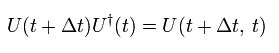( Because U means "retrogression" to time t from zero. )

Using Eq.36, Eq.35 can be expressed as
(Eq.37)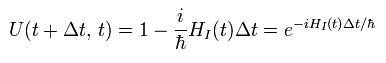Eq.37 is the infinitesimal time. When we connect U of Eq.37, as follows,
(Eq.38)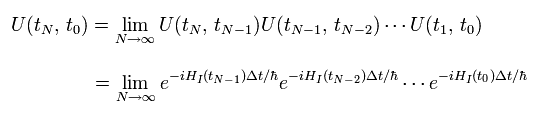Generally, the interaction Hamiltonian ( HI(t) ) doesn't commute when the times t are different.
Note that various U(t, t') of Eq.38 stand in time order, later on the left.

So we can express Eq.38 simply using the following time-ordering symbol T,
(Eq.39)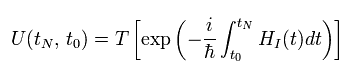Eq.39 means that when we remove this symbol T, each U(t, t') stand in time order like Eq.38 automatically.
( So we can exchange U(t, t') freely in symbol T, and connect them as one exponential function like Eq.39. )

### [ Green's function. ]

A propagator is the simplist form of Green functions, because it contains only two field operators ( 2 × φ ).
General form of Green function is
(Eq.40)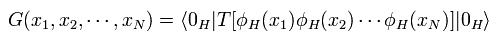where H means Heisenberg picture, which is explained in the upper section.

From Eq.31, the operators of Heisenberg and interaction pictures are
(Eq.41)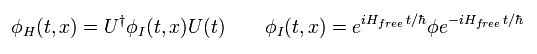where the unitary operator U(t) satisfies
(Eq.42)Substituting Eq.41 and Eq.42 into Eq.40,
(Eq.43)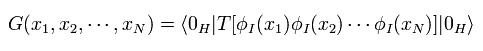In the time-ordering symple T, we can exchange the order of the operators freely, as I said in the upper section.

We define the vacuum energy is zero at time t = 0, as follows,
(Eq.44)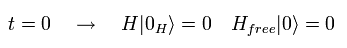(Caution: The vacuum of Heisenberg picture is different from the vacuum, because it contains infinite virtual particles including infinite momentum. )
From Eq.44,
(Eq.45)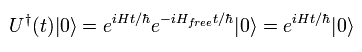The complete set of eigenfunctions in Heisenberg picture is
(Eq.46)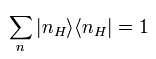Inserting Eq.46 into Eq.45,
(Eq.47)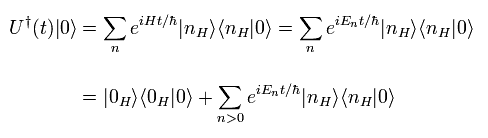where En > 0.
If we take the imaginary time of
(Eq.48)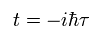when time → - ∞
(Eq.49)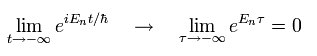So only zero energy of Heisenberg picture is left in Eq.47.
(This part is very difficult to imagine. But this "mathematical" world is QFT, as I said many times.)

From Eq.47 and Eq.49,
(Eq.50)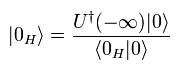In the same way (like from Eq.45 to Eq.50 ), we can get
(Eq.51)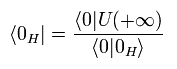From Eq.50 and Eq.51,
(Eq.52)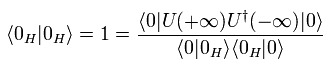so,
(Eq.53)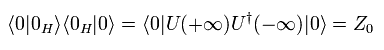Using Eq.50, Eq.51, and Eq.53, Green function of Eq.43 becomes
(Eq.54)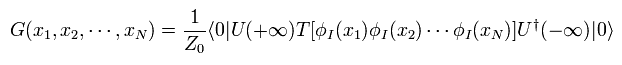As I said, if we insert U(t) operators into the time-ordering symple T, we can exchange the order of them freely, as follows,
(Eq.55)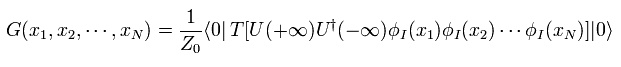Using Eq.36 - Eq.39, Eq.55 becomes
(Eq.56)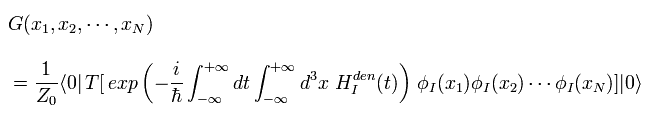where Hden means interaction Hamiltonian density. So Eq. 56 integrate it by space coordinates.

Z0 of Eq.53 means infinite disconnected diagrams ( vacuum bubbles ) contained in U(t).
So in Eq.56, disconnected vacuum bubbles of the numerator are eliminated by this denominator Z0.
As a result, it becomes sum of all diagrams connected to field operators (φ).

Eq.56 is the basic equations wich express Feynman diagrams. So this is very important.2011/1/22 updated. Feel free to link to this site.